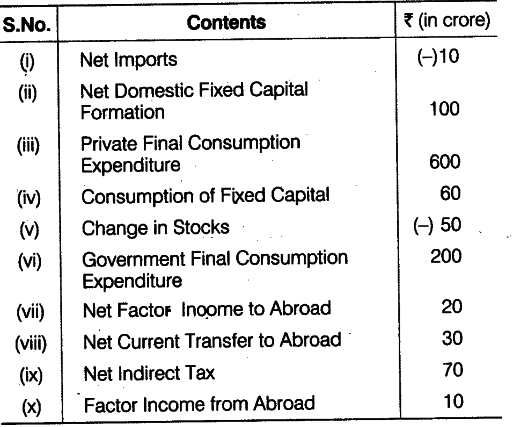# Find out the National Income

Find out:
(a) National Income
(b) Net National Disposable Income(a) \$GD{{P}_{MP}}\$ = Private Final Consumption Expenditure + Government Final Consumption Expenditure + Net Domestic Fixed Capital Formation + Change in Stocks

• Consumption of Fixed Capital - Net Imports
= 600 + 200 +100 + (- 50) + 60 - (-10) =970-50 =Rs. 920 crore
National Income (\$NN{{P}{FC}}\$) =
\$GD{{P}
{MP}}\$ - Net Factor Income to Abroad - Net Indirect Tax - Consumption of Fixed Capital
=920 - 20 - 70 - 60 = Rs. 770 crore
(b) Net National Disposable Income = \$NN{{P}_{FC}}\$ + Net Indirect Taxes - Net Current Transfer to Abroad =770+70-30 =840-30 =Rs. 810 crore